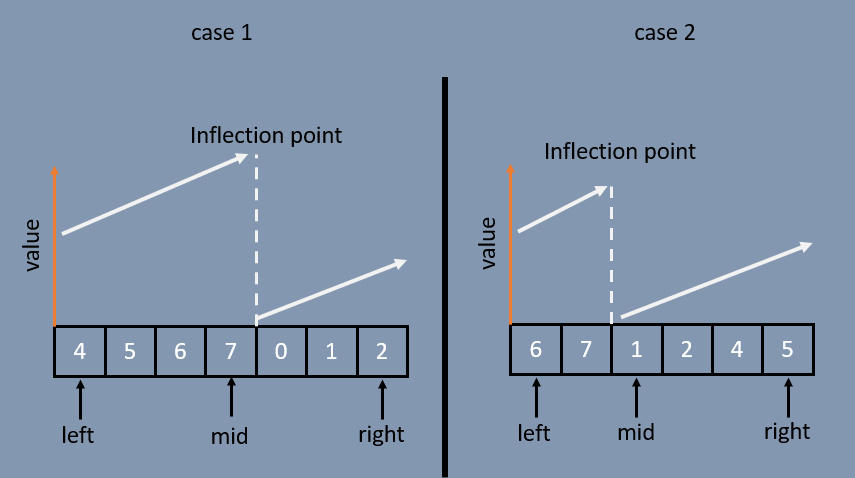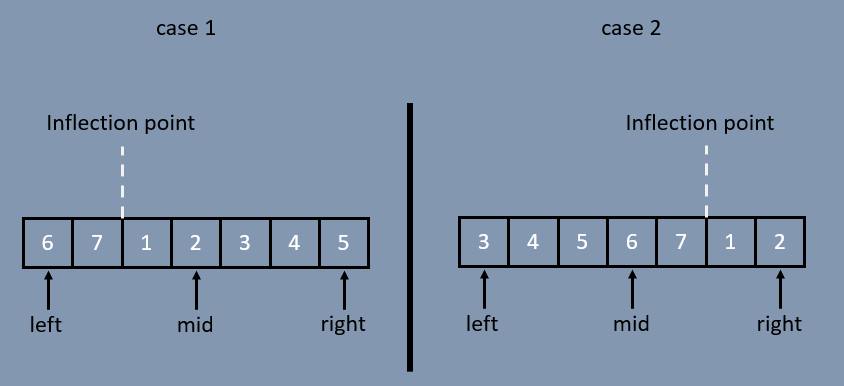# Leetcode 153 — Find Minimum in Rotated Sorted Array

Today’s article will cover and explain the optimal solution to Leetcode 153 — Find Minimum in Rotated Sorted Array.

# Problem Overview

Suppose an array of length `n` sorted in ascending order is rotated between `1` and `n` times. For example, the array `nums=[0,1,2,4,5,6,7]` might become:

`[4,5,6,7,0,1,2]` if it was rotated `4` times.

`[0,1,2,4,5,6,7]` if it was rotated `7` times.

Notice that rotating an array `[a,a,a, ... ,a[n-1]]` 1 time results in the array `[a[n-1], a, a, a, ... ,a[n-2]]`.

Given the sorted rotated array `nums` of unique elements, return the minimum element of this array. You must write an algorithm that runs in `O(log(n))` time.

Constraints:

`1 <= n <= 5000`

`-5000 <= nums[i] <= 5000`

`All the integers of nums are unique`

`nums is sorted and rotated between 1 and n times`

The constraints are important here, in this case they’re giving us some very useful information to make assumptions from. The obvious bit is handling the trivial case where `len(n) == 1` should be accounted for. Next, knowing all the elements are unique gives us assurance that there is a solution, and only 1 solution. Finally, we’re given information of another trivial case, one covered in the problem description; that is, if the array is rotated `n` times, it is equivalent to the original array. In otherwords, `sorted(nums) == rotated(sorted(nums), len(nums))` , and in that case we’d know the minimum number is `nums`.

# Example

`Input: nums = [3,4,5,1,2]Output: 1Explanation: The original array was [1,2,3,4,5] rotated 3 times.`

The example doesn’t really give us much. It’s mostly a sanity check.

# Solution Discussion

As I mentioned in my comments after the Problem Overview, there are two simple ‘base’ cases that we can get out of the way from the get go:

Let’s take a look at this for a second. If `nums` is a single element, it is by definition of being the only element, the minimum. Okay, case handled.

When `len(nums)>= 2` we now have to find the min. But what do we know from the overview? We know that the array is 1) sorted (strictly increasing left to right) and 2) rotated 1 to n (`n == len(nums)`) times, and rotating an array n times is equivalent to not rotating the array at all. So, given those bits of info, we check that case with `nums<nums[len(nums)-1]` .

## Modified Binary Search

With those two easy cases checked, we now know that our array is rotated in such a way that we’re going to need to iterate over it to find the min. This problem is set up to utilize a binary search. Divide and conquer this array.

Before we get to that point, I first want to point out a key concept to understanding the solution. When an array is sorted in ascending order (this case), the elements are strictly increasing. Meaning, `num[i] < num[i+1]` always. Once the array is rotated, there exists some point that violates that condition. For example: `[5, 6, 7, 1, 2, 3]` was rotated and at index `3` the array decreases before resuming it’s increasing behavior. The point where the array decreases, or ‘resets’, is the inflection point.

Then, let me pose a question to you: How do you identify the minimum value if middle was at one of these positions (for example):How do you determine the inflection point with these pointers?

This introduces us to how we identify that minimum value. Different from a normal binary search where we check `nums[mid]==target` , we need to check is mid on the inflection point, and if so, is it the minimum or the maximum? We do this with the following code:

So, we’ve figured out our equivalent to checking if `nums[mid]` is the target. Next, is how do we determine which side to divide and conquer to? In a normal binary search, we simply check `target < nums[mid]` to know if target is on the left or right side of the middle. In this problem, it is a bit different. Using the examples below, I pose this question: How do you determine which side to search?Using the 3 pointers, how do you decide which side to search next?

Let’s begin with case 2 because it is the easier of the 2 to follow. If you answered check `nums[left] < nums[mid]` you’d be correct! Notice, we know our array is only increasing on the left side by nature of `nums[mid]` remaining greater than all to it’s left.

In the same thought idea, case 1, checks if `nums[left] > nums[mid]` indicating that the inflection point is on the left hand side.

Note: You can also check if `nums < nums[mid]` because it implies the same property that what is on your left must be strictly and always increasing for the middle to be larger (property of the array being sorted).

Putting it all together, we have the following solution:

I hope you enjoyed the article! Thanks for reading!

--

--## Thomas Higginson

Software Engineer working on Cognitive EW capabilities, and human that enjoys making smiles. Welcome.